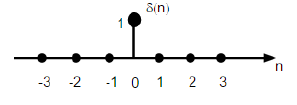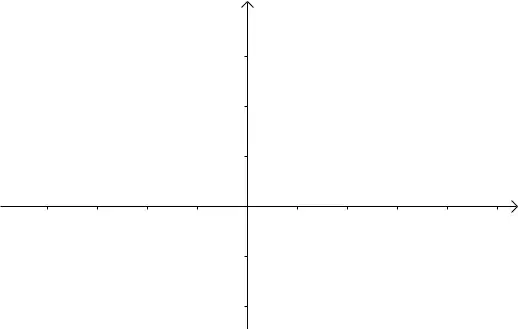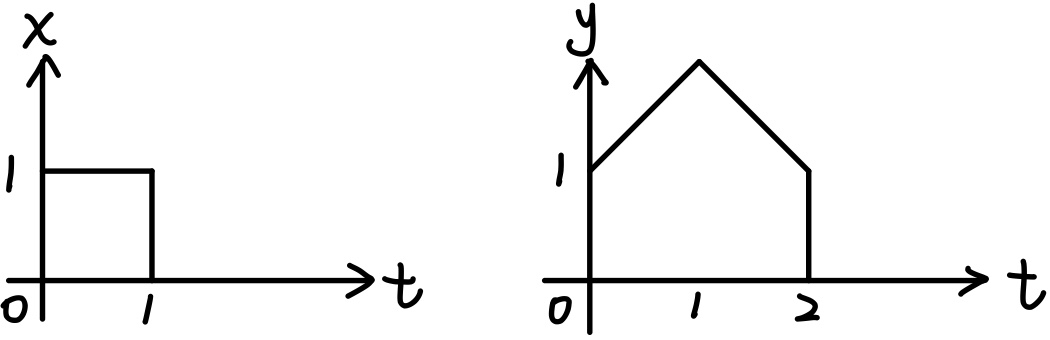# 卷积和与卷积积分

\begin{align*}\newcommand{\dif}{\mathop{}\!\mathrm{d}}\end{align*}

# 引入

## 卷积和1. 时不变性质：$\delta[n-k]\rightarrow h[n-k]$
2. 线性性：$x[k]\delta[n-k]\rightarrow x[k]h[n-k]$

# 卷积

f, g 的卷积记为 $f*g$# 卷积的性质

1. 交换律 Commutativity：$f_1*f_2=f_2*f_1$
Proof \text{Let } \sigma=t-\tau\\ \begin{align} f_1(t)*f_2(t)&=\int_{\infty}^{-\infty} f_1(t-\sigma)f_2(\sigma)(-\dif \sigma)\\ &=\int_{-\infty}^{\infty} f_2(\sigma)f_1(t-\sigma)\dif \sigma\\ &=f_2(t)*f_1(t) \end{align}
2. 结合律 Associativity：$(f_1*f_2)*f_3=f_1*(f_2*f_3)$
3. 分配律 Distributivity：$f_1*(f_2+f_3)=f_1*f_2+f_1*f_3$
4. Duration：Let the signals $f_1(t)$ and $f_2(t)$ have durations, respectively, defined by the time intervals $[t_1, T_1]$ and $[t_2,T_2]$ then

$% $

Proof $$(f_1*f_2)(t)=\int_{-\infty}^{\infty} f_1(\tau)f_2(t-\tau) \dif \tau\\ \because \tau\in [t_1,T_1],\; t-\tau\in [t_2, T_2]\\ \therefore t_1 \leq \tau \leq T_1,\; t_1+t_2\leq t \leq T_1+T_2\\$$ $$(f_2*f_1)(t)=\int_{-\infty}^{\infty} f_2(\tau)f_1(t-\tau) \dif \tau\\ \because \tau\in [t_2,T_2],\; t-\tau\in [t_1, T_1]\\ \therefore t_2 \leq \tau \leq T_2,\; t_1+t_2\leq t \leq T_1+T_2\\$$ $$综上，\frac{t_1+t_2}{2}\leq \tau \leq\frac{T_1+T_2}{2}\\ t_1+t_2\leq t \leq T_1+T_2$$
5. 时移 Time Shifting：Let $f(t)=f_1(t)*f_2(t)$. Then, convolutions of shifted signals are given by $f(t-\sigma_1)=f_1(t-\sigma_1)*f_2(t)\\ f(t-\sigma_2)=f_1(t)*f_2(t-\sigma_2)\\ f(t-\sigma_1-\sigma_2)=f_1(t-\sigma_1)*f_2(t-\sigma_2)$
6. 微分：$\frac{\dif}{\dif t} [f(t)*g(t)]=\left[ \frac{\dif}{\dif t} f(t) \right]*g(t)$ $=f(t)* \left[ \frac{\dif}{\dif t} g(t) \right]$（不是加）
7. 积分：$\int_{-\infty}^t f(\tau)*g(\tau) \dif \tau$ $=\int_{-\infty}^t f(\tau)\dif \tau * g(\tau)$ $=f(\tau)*\int_{-\infty}^t g(\tau)\dif \tau$（不是加）
8. 多重微积分：$f(t)^{(m)}*g(t)^{(n)}=[f(t)*g(t)]^{(m+n)}$

• 利用交换律，使得简单地函数处在 $f(t-\tau)$ 的位置。（合理选择作为“平移”的函数）

$$e^{-t}u(t)*\sin(t)=\int_{-\infty}^{\infty} e^{-(t-\tau)} u(t-\tau)\sin(\tau) \dif \tau\\ =\int_{-\infty}^{\infty} e^{-(\tau)} u(\tau)\sin(t-\tau) \dif \tau$$

\begin{align} e^{-t}u(t)*\sin(t)&=\int_{-\infty}^{\infty} e^{-(t-\tau)} u(t-\tau)\sin(\tau) \dif \tau\\ &=e^{-t}\int_{-\infty}^t e^\tau \sin(\tau)\dif \tau\\ &=e^{-t}\left[ \frac{e^t}{2}\left( \sin t -\cos t \right)-0 \right]\\ &=\frac{1}{2}(\sin t -\cos t ) \end{align}

\begin{align} \because \int_0^\infty e^{-\tau}\sin(t-\tau)\dif \tau &= \int_0^\infty e^{-\tau}[\sin t\cos\tau-\cos t\sin\tau]\dif \tau\\ \therefore e^{-t}u(t)*\sin(t)&=\int_{-\infty}^{\infty} e^{-(\tau)} u(\tau)\sin(t-\tau) \dif \tau\\ &=\sin(t)\int_0^\infty e^{-\tau} \cos(\tau)\dif \tau -\cos(t)\int_0^\infty e^{-\tau}\sin(\tau) \dif \tau\\ &=\sin(t)\left[ 0-\frac{1}{2}(-\cos 0+\sin0) \right]-\cos(t)\left[0-\frac{1}{2}(-\sin 0-\cos0)\right]\\ &=\frac{1}{2}(\sin t -\cos t ) \end{align}

• 利用微分、积分化简折线形的信号

$$x(t)=u(t)-u(t-1)\\ y(t)= \begin{cases} 1+x & 0\leq x \lt 1\\ 3-x & 1\leq x \lt 2 \end{cases}$$$$\frac{\dif y(t)}{\dif t}= \begin{cases} 1 & 0\leq x \lt 1\\ -1 & 1\leq x \lt 2 \end{cases}$$

$$\int^{t}_{-\infty} x(t)*\frac{\dif y(t)}{\dif t} \dif t=x(t)*\int^{t}_{-\infty} \frac{\dif y(t)}{\dif t} \dif t = x(t)*y(t)$$